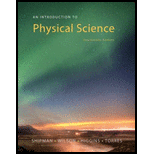Chapter 1, Problem 2SAAn Introduction to Physical Science

14th Edition
James Shipman + 3 others
ISBN: 9781305079137

Solutions

Chapter
SectionAn Introduction to Physical Science

14th Edition
James Shipman + 3 others
ISBN: 9781305079137
Textbook Problem

What are the five major divisions of physical science?

To determine
Different types of physical science.

Explanation

The physical science involves the study of nonliving matter. There are five division of physical science.

Physics is concerned with the basic principles, concepts of matter and energy.

Chemistry deals with the structure, reactions of matter and composition.

Astronomy is the study of the universe, which is the sum of all matter, space, energy and time...

Still sussing out bartleby?

Check out a sample textbook solution.

See a sample solution

The Solution to Your Study Problems

Bartleby provides explanations to thousands of textbook problems written by our experts, many with advanced degrees!

Get Started